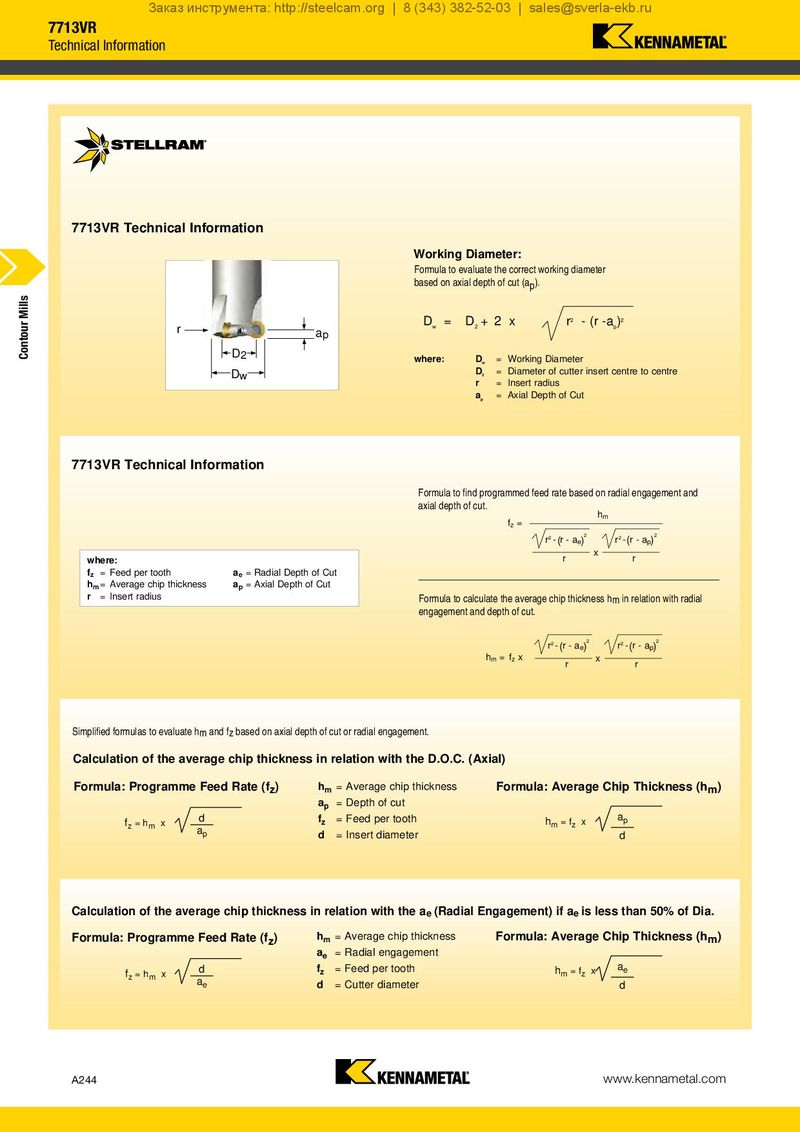Заказ инструмента: http://steelcam.org | 8 (343) 382-52-03 | [email protected] 7713VR Technical Information 7713VR Technical Information Working Diameter: Formula to evaluate the correct working diameter based on axial depth of cut (ap). Contour Mills r D w = D+2 2 x r2 - (r -a )2 p ap D2 where: D w = Working Diameter Dw D 2 = Diameter of cutter insert centre to centre r = Insert radius a p = Axial Depth of Cut 7713VR Technical Information Formula to find programmed feed rate based on radial engagement and axial depth of cut. hm fz = r2 - (r - ae)2 r2 - (r - ap)2 where: r x r fz = Feed per tooth ae = Radial Depth of Cut hm = Average chip thickness ap = Axial Depth of Cut r = Insert radius Formula to calculate the average chip thickness hm in relation with radial engagement and depth of cut. r2 - (r - ae)2 r2 - (r - ap)2 hm = fz x r x r Simplified formulas to evaluate hm and fz based on axial depth of cut or radial engagement. Calculation of the average chip thickness in relation with the D.O.C. (Axial) Formula: Programme Feed Rate (fz) hm = Average chip thickness Formula: Average Chip Thickness (hm) ap = Depth of cut fz = hm x d fz = Feed per tooth hm = fz x ap ap d = Insert diameter d Calculation of the average chip thickness in relation with the ae (Radial Engagement) if ae is less than 50% of Dia. Formula: Programme Feed Rate (fz) hm = Average chip thickness Formula: Average Chip Thickness (hm) ae = Radial engagement fz = hm x d fz = Feed per tooth hm = fz x ae ae d = Cutter diameter d A244 www.kennametal.com